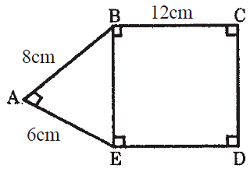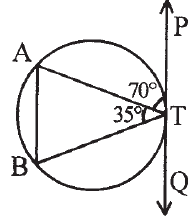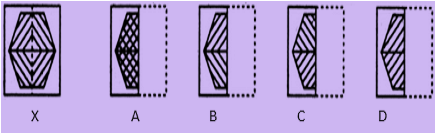# CREST Olympiads - CMO PDF Sample Papers for Class 10

Resources:

Class 10 sample paper & practice questions for CREST Mathematics Olympiad (CMO) level 1 are given below. Syllabus for level 1 is also mentioned for these exams. You can refer these sample paper & quiz for preparing for the CMO exam.Q.1 Q.2 Q.3 Q.4 Q.5 Q.6 Q.7 Q.8 Q.9 Q.10
 Q.1 Which prime numbers will be repeatedly multiplied in prime factorization of 3200? a) 2 and 5 b) 4 and 2 c) 2 and 6 d) 5 and 8
 Q.2 Find the value of x which satisfies the equation: x + 4/x = -4; x ≠ 0 a) 2 b) -2 c) 3 d) -3
 Q.3 In figure AB = 8 cm, BC = 12 cm and AE = 6 cm. Find area of the rectangle BCDE.a) 120 cm² b) 190 cm² c) 220 cm² d) 150 cm²
 Q.4 What is the relation between my daughter and my mother's sister's father-in-law? a) father b) grandfather c) great grandchild d) niece
 Q.5 Find out from the four alternatives as to how the pattern would appear when the transparent sheet is folded at the dotted line.a) A b) B c) C d) D
 Q.6 Gunjan walks 12m due East and then 5m due North. At what distance is Gunjan from the starting point? a) 10m b) 13m c) 11m d) 15m
 Q.7 What will be the lateral surface area of a cylinder (in terms of radius r) whose height is twice of its radius? a) 2πr3 b) 4πr2 c) 6πr2 d) 8πr2
 Q.8 First term of an A.P. is –2 and 10th term is 28. What is the value of d (common difference)? a) 10/3 b) 10/9 c) 10/6 d) 10/4
 Q.9 Find ∠BTQ?a) 30⁰ b) 45⁰ c) 75⁰ d) 90⁰
 Q.10 Find out from the four alternatives as to how the pattern would appear when the transparent sheet is folded at the dotted line.a) B b) D c) C d) ASample PDF of CREST Olympiads - CREST Mathematics Olympiad (CMO) PDF Sample Papers for Class 10: